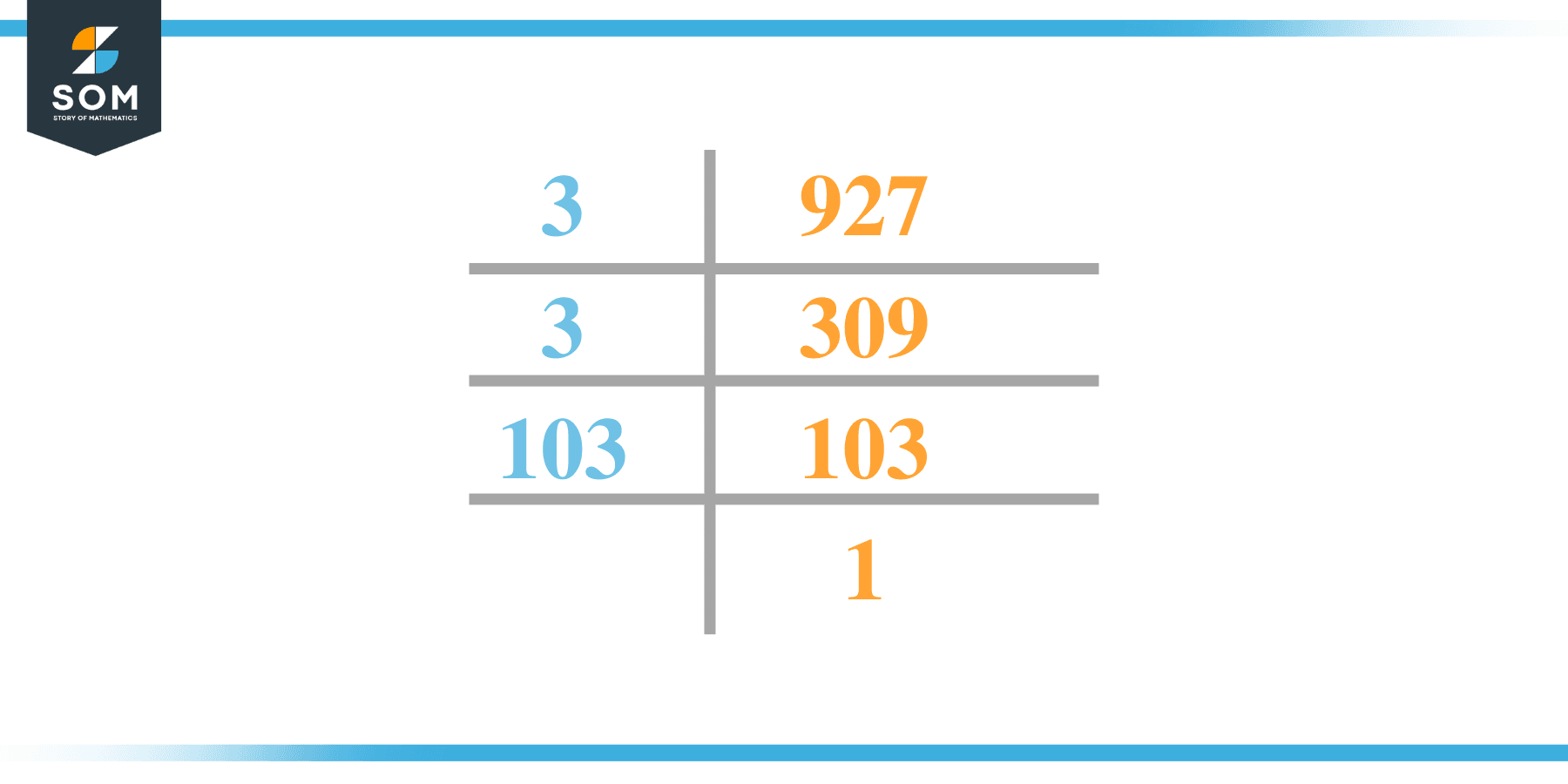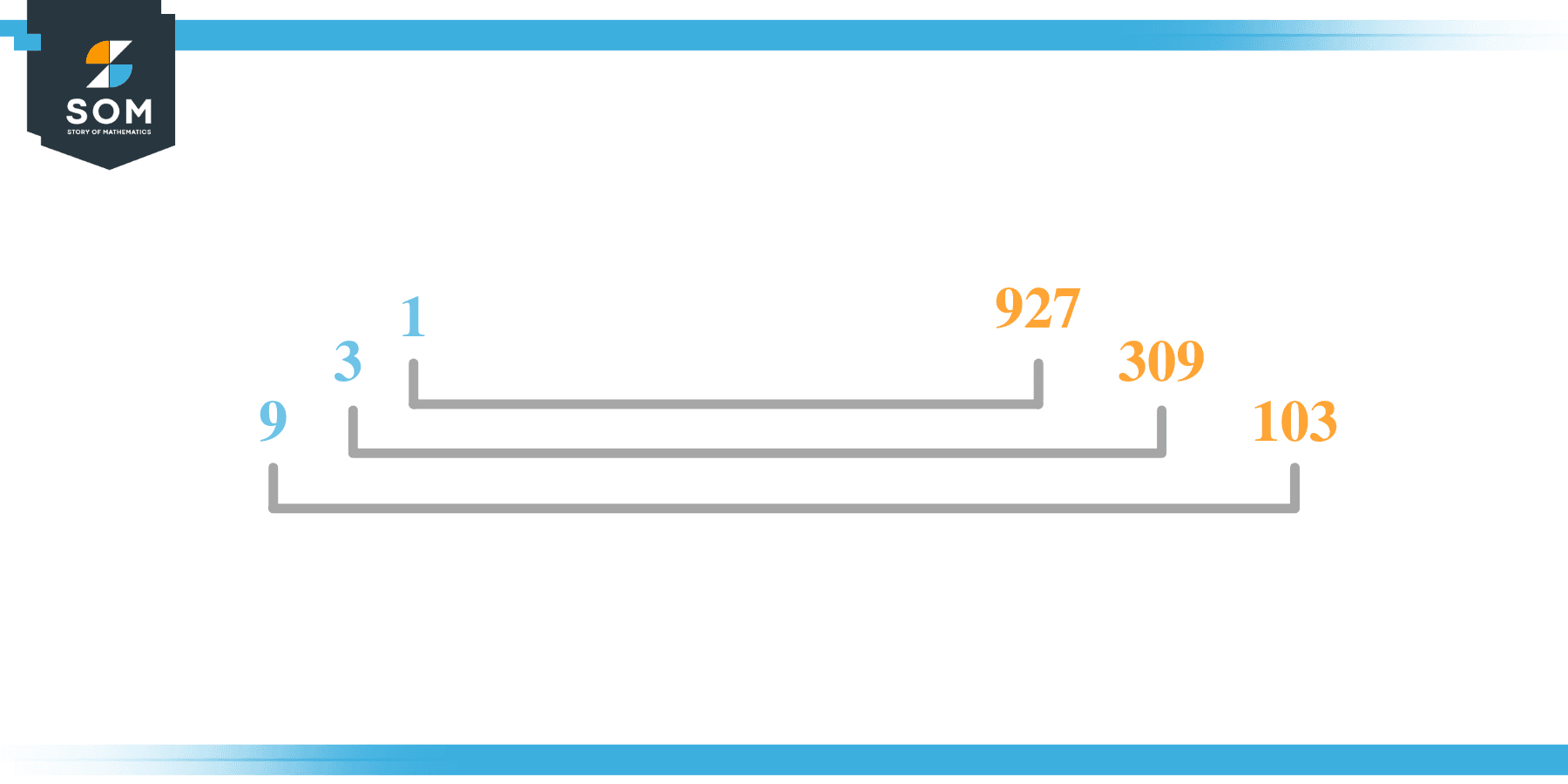# Factors of 927: Prime Factorization, Methods, and Examples

Let us see the factors of number 927 in this solution along with prime factors and examples. As the number 927 is concerned so firstly it becomes an odd number because not completely divisible by 2.Also, it contains more than two factors which we see below so it is a composite number.

### Factors of 927

Here are the factors of number 927.

Factors of 927: 1, 3, 9, 103, 309, and 927

### Negative Factors of 927

The negative factors of 927 are similar to its positive aspects, just with a negative sign.

Negative Factors of 927: -1, -3, -9, -103, -309, and -927

### Prime Factorization of 927

The prime factorization of 927 is the way of expressing its prime factors in the product form.

Prime Factorization: 3 x 3 x 103

In this article, we will learn about the factors of 927 and how to find them using various techniques such as upside-down division, prime factorization, and factor tree.

## What Are the Factors of 927?

The factors of 927 are 1, 3, 9, 103, 309, and 927. These numbers are the factors as they do not leave any remainder when divided by 927.

The factors of 927 are classified as prime numbers and composite numbers. The prime factors of the number 927 can be determined using the prime factorization technique.

## How To Find the Factors of 927?

You can find the factors of 927 by using the rules of divisibility. The divisibility rule states that any number, when divided by any other natural number, is said to be divisible by the number if the quotient is the whole number and the resulting remainder is zero.

To find the factors of 927, create a list containing the numbers that are exactly divisible by 927 with zero remainders. One important thing to note is that 1 and 927 are the 927’s factors as every natural number has 1 and the number itself as its factor.

1 is also called the universal factor of every number. The factors of 927 are determined as follows:

$\dfrac{927}{1} = 927$

$\dfrac{927}{3} = 309$

$\dfrac{927}{9} = 103$

$\dfrac{927}{103} = 9$

$\dfrac{927}{309} = 3$

$\dfrac{927}{927} = 1$

Therefore, 1, 3, 9, 103, 309, and 927 are the factors of 927.

### Total Number of Factors of 927

For 927, there are six positive factors and six negative ones. So in total, there are twelve factors of 927.

To find the total number of factors of the given number, follow the procedure mentioned below:

1. Find the factorization/prime factorization of the given number.
2. Demonstrate the prime factorization of the number in the form of exponent form.
3. Add 1 to each of the exponents of the prime factor.
4. Now, multiply the resulting exponents together. This obtained product is equivalent to the total number of factors of the given number.

By following this procedure, the total number of factors of 927 is given as:

Factorization of 927 is 3 x 3 x 103.

The exponent of 3 and 103 is 2,1.

Adding 1 to each and multiplying them together results in six.

Therefore, the total number of factors of 927 is twelve. Six are positive, and six factors are negative.

### Important Notes

Here are some essential points that must be considered while finding the factors of any given number:

• The factor of any given number must be a whole number.
• The factors of the number cannot be in the form of decimals or fractions.
• Factors can be positive as well as negative.
• Negative factors are the additive inverse of the positive factors of a given number.
• The factor of a number cannot be greater than that number.
• Every even number has 2 as its prime factor, the smallest prime factor.

## Factors of 927 by Prime FactorizationThe number 927 is a composite. Prime factorization is a valuable technique for finding the number’s prime factors and expressing the number as the product of its prime factors.

Before finding the factors of 927 using prime factorization, let us find out what prime factors are. Prime factors are the factors of any given number that are only divisible by 1 and themselves.

To start the prime factorization of 927, start dividing by its most minor prime factor. First, determine that the given number is either even or odd. If it is an even number, then 2 will be the smallest prime factor.

Continue splitting the quotient obtained until 1 is received as the quotient. The prime factorization of 927 can be expressed as:

927 = 3 x 3 x 103

## Factors of 927 in PairsThe factor pairs are the duplet of numbers that, when multiplied together, result in the factorized number. Factor pairs can be more than one depending on the total number of factors given.

For 927, the factor pairs can be found as:

1 x 927 = 927

3 x 309 = 927

9 x 103 = 927

The possible factor pairs of 927 are given as (1, 927), (3, 309), and (9, 103).

All these numbers in pairs, when multiplied, give 927 as the product.

The negative factor pairs of 927 are given as:

-1 x -927 = 927

-3 x -309 = 927

-9 x -103 = 927

It is important to note that in negative factor pairs, the minus sign has been multiplied by the minus sign, due to which the resulting product is the original positive number. Therefore, -1, -3, -9, -103, -309, and -927 are called negative factors of 927.

The list of all the factors of 927, including positive as well as negative numbers, is given below.

Factor list of 927: 1, -1, 3, -3, 9, -9, 103, -103, 309, -309, 927, and -927

## Factors of 927 Solved Examples

To better understand the concept of factors, let’s solve some examples.

### Example 1

How many factors of 927 are there?

### Solution

The total number of Factors of 927 is six.

Factors of 927 are 1, 3, 9, 103, 309, and 927.

### Example 2

Find the factors of 927 using prime factorization.

### Solution

The prime factorization of 927 is given as:

927 $\div$ 3 = 309

309 $\div$ 3 = 103

103 $\div$ 103 = 1

So the prime factorization of 927 can be written as:

3 x 3 x 103 = 927